output.to from Sideway
Number Theory

Factorization

Pollard's p-1 Method

Draft for Information Only

# Content

``` Pollard's p-1 Method     Pollard's P-1 Methed by PowerSmooth Number     Pollard's P-1 Methed by PowerSmooth Number Example 2```

# Pollard's p-1 Method

Pollard's p-1 method is a prime factorization algorithm discovered by John Pollard in 1974. Limited by the algorithm, the Pollard's p-1 method is only work for integers with specific factors.

If the number n has a prime factor p such that p-1 can be expressed in terms of a product of primes, the finding of prime factor p is based on the selected boundary B of the B-powersmooth number.  The algorithm fails when the selected boundary B is smaller than the B-powersmooth number of p-1.

#### Pollard's P-1 Methed by PowerSmooth Number

##### Pollard's P-1 Methed by PowerSmooth Number Example 2

For example: n=667=p*q=23*29; let B=5 imply

Integer B-smooth number Prime Factors number
k 5 22*31*51 = 4*3*5 60

Therefore for B=5, k5 or (p5-1)m5 is equal to 60.

###### Fermat's Little Theorem

let a=2, by Fermat's little theorem, let p be one of the prime factors of n, imply p idivides ak-1.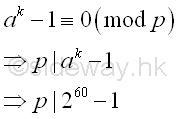###### Greatest Common Divisor

Since ak-1 is a very large number, before finding the greatest common divisor of n and ak-1,  ak-1 can be raised to the high power modulo n. Imply

Using squarings modulo

base  number; a=2; k=60; n=667
ak base 10 260
ai base 10 21  = 21 ≡ 2 (mod 667)
22 = 22 ≡ 4 (mod 667)
24 = 42 ≡ 16 (mod 667)
28 = 162 ≡ 256 (mod 667)
216 = 2562 ≡ 170 (mod 667)
232 = 1702 ≡ 219 (mod 667)
ak base 10 232+16+8+4
ak base 10 232*216*28*24
ak base 10 219*170*256*16 ≡ 538 (mod 667)

and using the residue to calculate the greatest common divisor. Imply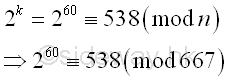The greatest common divisor of n and ak-1 is

Using Euclid's algorithm

ak-1 n
260-1 667
538-1 667
537 667-537=130
537-4*130=17 130
17 130-7*17=11
17-11=6 11
6 11-6=5
6-5=1 5
1 5-5*1=0
1 0

Imply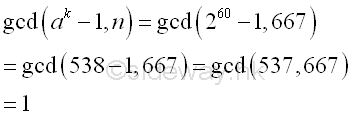The algorithm fails because the greatest common divisor of n and ak-1 is equal to 1. The number n does not have prime divisor p with p-1 is 5-powersmooth. Therefore the bound B equals to 5 fails, a larger bound B' should be used.

Let B=7,  imply

Integer B-powersmooth number Prime Factors number
k 22*31*51*71 = 4*3*5*7 60 * 7 = 420

Therefore for B=7, k7 or (p7-1)m7 is equal to 60*7 = 420. Imply  k7 =  k5 * 7. And  the new ak can be expressed as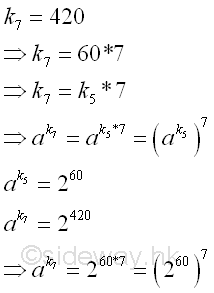And ak can be raised to the high power modulo n, imply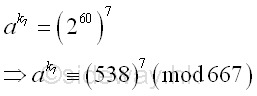Using squarings modulo

base  number; a=538; k=7*; n=667
ak base 10 5387 (mod 667)
ai base 10 5381  = 5381 ≡ 538 (mod 667)
5382 = 5382 ≡ 633 (mod 667)
5384 = 6332 ≡ 489 (mod 667)
ak base 10 5384+2+1 (mod 667)
ak base 10 5384*5382*5381 (mod 667)
ak base 10 489*633*538 ≡ 349 (mod 667)

and using the residue to calculate the greatest common divisor. Imply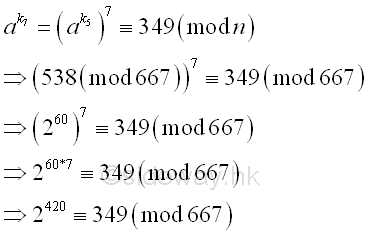The greatest common divisor of n and ak-1 is

Using Euclid's algorithm

ak-1 n
2420-1 667
349-1 667
348 667-348=319
348-319=29 319
29 319-11*29=0
29 0

Imply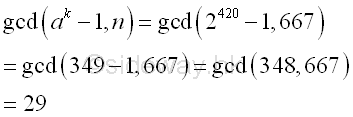Integer 29, the greatest common divisor of n and ak-1 is also the prime divisor of n. And p-1 is  7-powersmooth.

Integer B-powersmooth number Prime Factors number
p-1 7 22*71 28
k 7 22*31*51*71 = 4*3*5*7 420
k/(p-1)   20*31*51*70 15

©sideway

ID: 120500010 Last Updated: 2012/5/17 Revision:Home (5)

Business

Management

HBR (3)

Information

Recreation

Hobbies (7)

Culture

Chinese (1097)

English (336)

Reference (66)

Computer

Hardware (149)

Software

Application (187)

Digitization (24)

Numeric (19)

Programming

Web (648)CSS (SC)

ASP.NET (SC)

HTML

Knowledge Base

Common Color (SC)

Html 401 Special (SC)

OS (389)

MS Windows

Windows10 (SC)

.NET Framework (SC)

DeskTop (7)

Knowledge

Mathematics

Formulas (8)

Number Theory (206)

Algebra (20)

Trigonometry (18)

Geometry (18)

Calculus (67)

Complex Analysis (21)

Engineering

Tables (8)

Mechanical

Mechanics (1)

Rigid Bodies

Statics (92)

Dynamics (37)

Fluid (5)

Control

Acoustics (19)

Biology (1)

Geography (1)

Copyright © 2000-2019 Sideway . All rights reserved Disclaimers last modified on 10 Feb 2019